Select Page

# Binary Operators in Relational Algebra

All those Operators which operate on two operands are known as Binary operators.

## Types of Binary Operators

There are 3 types of binary operators which are given below

1. Union Operators
2. Cross Product
3. Difference operator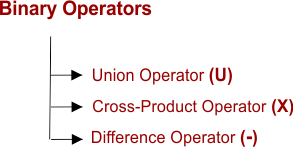### 1. Union Operator (∪)

Let A and B be two relations.

Then

• A ∪ B is the set of all tuples belonging to either A or B or both.
• In A ∪ B, duplicates are automatically removed.
• Union operation is both commutative and associative.

#### Example:

Consider the following two relations A and B,

Relation A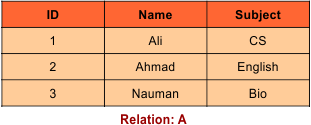And Relation B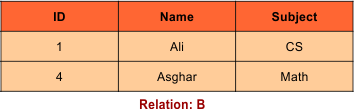Then, A ∪ B is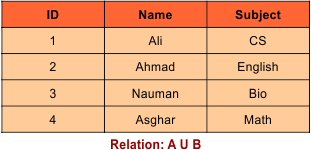### 2. Cartesian/CROSS Product

Cross product is a way of combining two tables. The resulting table will contains each of the attributes in both tables being combined.

It is denoted by ‘✕’ symbol

If A and B are two tables and needs to a cross product between them then it will be given below

A ✕ B, Where A and B are the two tables,

#### Example:

Consider following tables

“Student” Table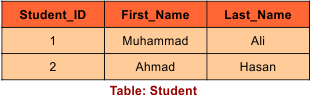And “Student_Detail” TableOn applying CROSS PRODUCT on STUDENT and Student_Detail tables is given below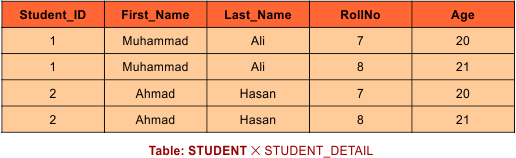### Important Terms

The cardinality (Number of Tuples/Rows):
Number of Tuples after Cross product = Rows of A table + Rows of B table

Degree (No of Columns)
Number of columns after Cross product = columns of A table  X columns of B table

In the above table We can see that the number of tuples (Rows) in STUDENT relation is 2, and the number of tuples in Student_DETAIL table is 2.

• So the number of tuples in the resulting table after performing CROSS PRODUCT is 2 x 2 = 4.
• Number of columns will be the sum of total columns in both tables so, 3+2=5

### 3. Difference Operator (-)

Let A and B be two relations.

Then

• A – B is the set of all tuples belonging to A and not to B.
• In A – B, duplicates are automatically removed.
• Difference operation is associative but not commutative.

#### Example

Consider the following two relations A and B

Relation ARelation BThen, A – B is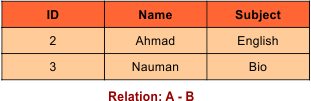Help Other’s By Sharing…